# 博客

## 集训队互测 2021 Round #2 题解

2021-01-23 18:00:50 By rushcheyo

### 逛公园

from Lagoon

#### 广义串并联图

1、对于删 $1$ 度点操作：建立一个新的节点 $v'$，将被删除点 $x$，与其相连的边 $a$ 和 $a$ 的另一个端点 $z$ 对应的树上节点 $v_x,v_a,v_z$ 的父亲设为 $v'$，然后将点 $z$ 对应的树上节点设为 $v'$。

2、对于叠合重边操作：建立一个新的节点 $v'$，将两条重边 $a,b$ 对应的树上节点 $v_a,v_b$ 的父亲设为 $v'$，然后将合并后的边对应的树上节点设为 $v'$。

3、对于缩 $2$ 度点操作：建立一个新的节点 $v'$，将被删除点 $x$ 和与其相连的两条边 $a,b$ 对应的树上节点 $v_x,v_a,v_b$ 的父亲设为 $v'$，然后将建立的新边对应的树上节点设为 $v'$。

#### 算法五

1、如果点 $u$ 在图上对应的是一个点，那么对于初始图 $G$，如果图上的一个点或一条边对应的树上叶子节点在点 $u$ 的子树中，那么这个点或边属于 $G_u'$。

2、如果点 $u$ 在图上对应的是一条边，设这条边为 $(x,y)$，那么对于初始图 $G$，如果图上的一个点或一条边对应的树上叶子节点在点 $u$ 的子树中，那么这个点或边属于 $G'_u$，且点 $x$ 和点 $y$ 也属于 $G'_u$。

#### 算法六

$$dis(x,y)=\min\{dis(x,p_1)+dis(y,p_1),dis(x,p_2)+dis(y,p_2)\}$$

### 快递公司

from shiliangzhi

#### 测试点一

$n=5$ 应该非常好想，显然两个五元环可以直接拼出完全图。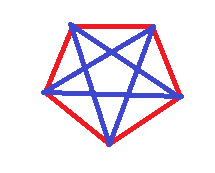#### 测试点二

$n=8$ 时，不难证明其至少需要 $3$ 种颜色（具体证明见下文）。

#### 测试点三

##### 测试点三--算法三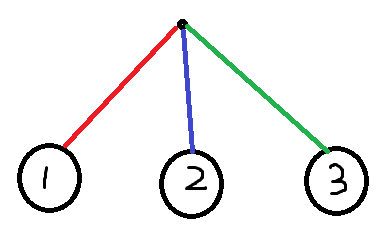#### 测试点六、七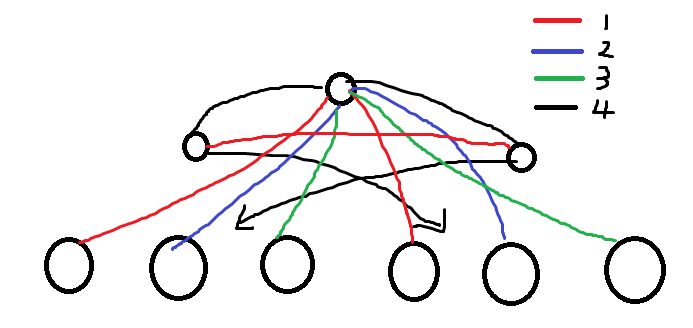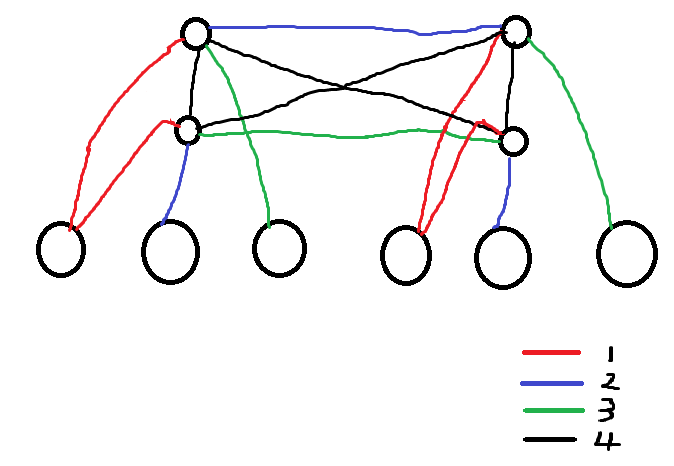#### 测试点八、九、十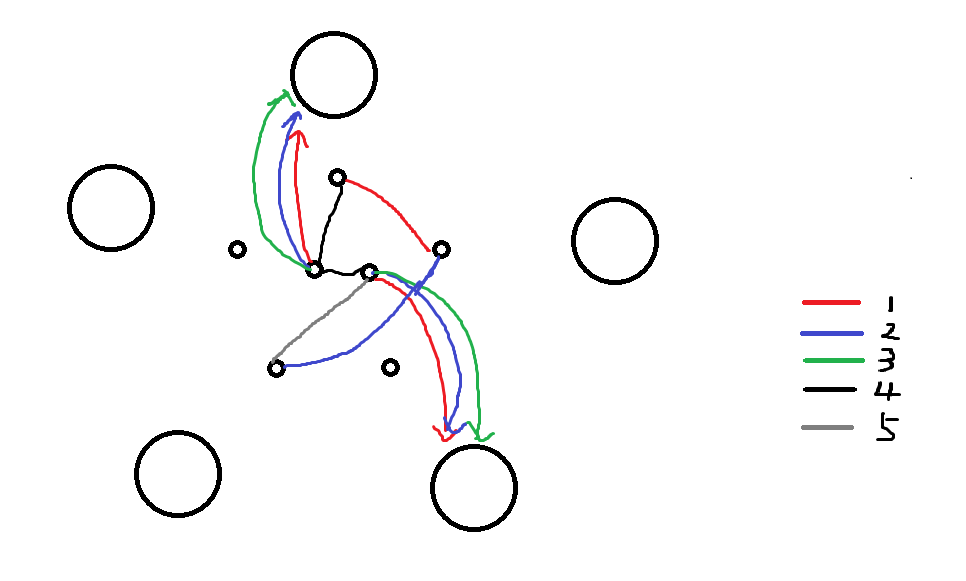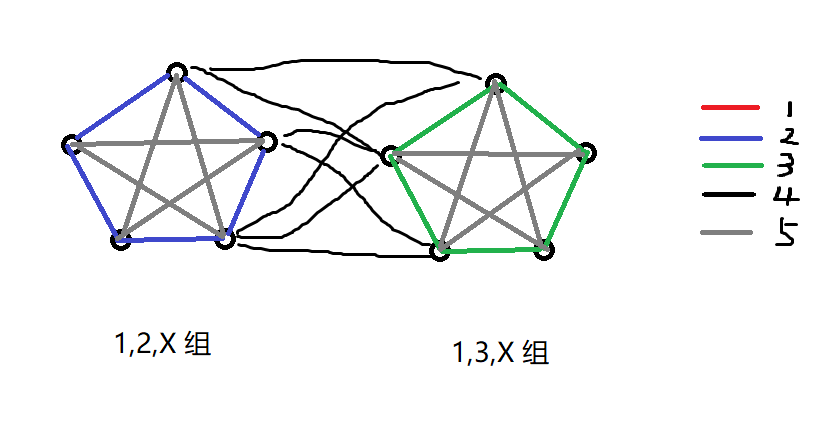### 小 C 的比赛

from rushcheyo

UPD：已经被 hos_lyric 解决，命题错误，反例如下：

 441 -441 106 -531 53 664 -901 -123 -254 75 404 629 -71 408 

1. $\{1,2\}$ 或 $\{-1,-2\}$，任意排布即可取到 $r$；

2. $\{-1,2\}$，我们将尽可能多的 $\texttt{2 -1 -1}$ 放在序列开头；之后剩下两种情况：

1. 全是 $-1$ 或全是 $2$，则取到了 $r$；

2. 除了一个 $-1$ 外全是 $2$，随意排布（但除非 $n=2$，否则 $-1$ 不能放在序列两端）即取到 $r$。

3. $\{-2,1\}$，用 2. 中的类似办法，但此时 $\texttt{1 1 -2}$ 中的 $\texttt{1 1}$ 可能对答案也有贡献，需特判一下答案是否为 $1$（此时 $1$ 和负数交替，也符合猜想）。

## 评论

skip2004

Lagoon

peehs_moorhsum
B题有以下简洁解法： 如果想把Kn用a种颜色染色使得没有同色三角形，可以先每个点随机染色。然后按随机顺序遍历所有边，每条边考虑固定其余边颜色后，该边选择什么颜色可以令同色三角形个数最少，在这样的颜色中随机选一个·。一直遍历直到同色三角形个数减为0个。可以在5s内跑出最大的点，并且很容易将题中的界加强。 更多类似解法的应用题可以参见俺的集训队论文（
skyline
B题简单解法(sub4-10) 给1~n-1染色，使得若x,y同色且x+y=z，则z是另一种颜色 x和y城市之间的公司为abs(x-y)的颜色 染色方法搜索即可，半分钟能出解 现场的时候我输错了参数，导致少了一位，以为后三个sub是4色的，所以没过= =
memset0

Myson_Zyh
【该评论涉嫌辱骂他人，已被管理员隐藏】